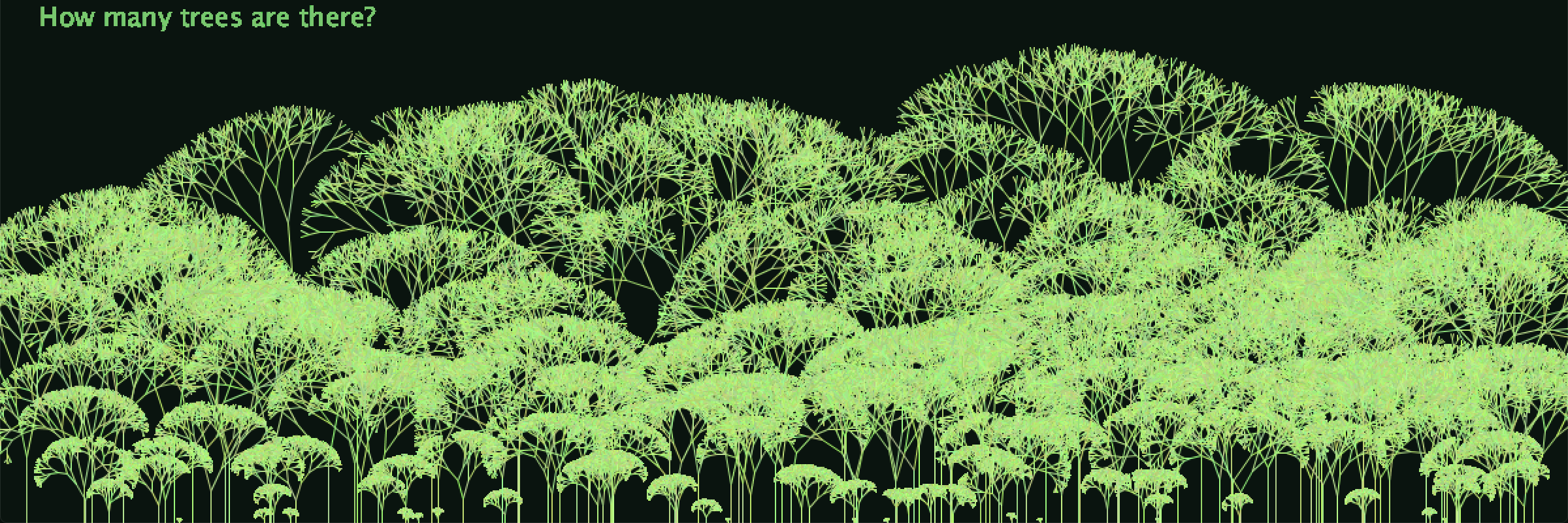# The trees of the world: Data VisualizationThis is how many trees there are(or at least how many there were in 2016).

I decided to do a data visualization of the percentage of land area covered by forest per country. The taller the tree, the bigger the percentage.

First I created a branch object, which I made into an array in my tree function, which I then make an array of in my draw function.

```ArrayList<Tree> trees;

float count = 0;
int data[];
Tree tree;
void setup() {
// I want to do three things,
//one create one tree for each point of data,
//two, make each tree's height dependent on the value of the data point
//three, be able to switch between data sets with a keypress. (less important)
size(1200, 400);
trees = new ArrayList<Tree>();
//if (mousePressed){
//}
//else {
//}
int[] data = new int[forest.length];

for(int i = 0; i < forest.length; i++){
data[i] = int(forest[i]);
}

for (int i = 0; i < data.length; i++) {
int x = int(random(20, width-20));
int y = int(random(height+4,height-4));
tree = new Tree(data[i], x, height );//enter height and position of tree
}
//float trunk = 50;
//float trunk = data.length;
}

void draw() {
background(10, 20, 15);
for (Tree tree : trees) {
tree.update();
tree.show();
fill(120,200, 110);
textSize(20);
text("How many trees are there?", 30, 20);

}
}```
```class Tree {
ArrayList<Branch> branches;
int trunk, x, y, count;

Tree(int trunk, int x, int y) {
branches = new ArrayList<Branch>();
this.trunk = trunk;
this.x = x;
this.y = y;
this.count = 0;

PVector a =  new PVector (x, y);
PVector b = new PVector (x, y-trunk);
Branch root = new Branch(a, b);
}

void update() {
if (count < 9) {
for (int i = branches.size() -1; i >= 0; i--) {
Branch current = branches.get(i);
//if the current Branch has no children: add them
if (!current.finished) {
}
//now that Branch has children
current.finished = true;
}
count ++;
}
}

void show() {
for (int i = 0; i < branches.size(); i++) {
branches.get(i).update();
}
}
}
```
```class Branch{

PVector start;
PVector end;
boolean finished = false;

Branch(PVector start,PVector  end){

this.start = start;
this.end = end;
}

void update() {

stroke(random(150,200),random(200,255),random(100,150));
line(start.x, start.y, end.x, end.y);

}

Branch branchA(){
PVector direction = PVector.sub(end, start);
direction.rotate(PI / random(4,20));
direction.mult(0.75);
Branch b = new Branch(end, newEnd);
return b;
}

Branch branchB(){
PVector dir = PVector.sub(end, start);
dir.rotate(- PI / random(4,20));
dir.mult(0.75);